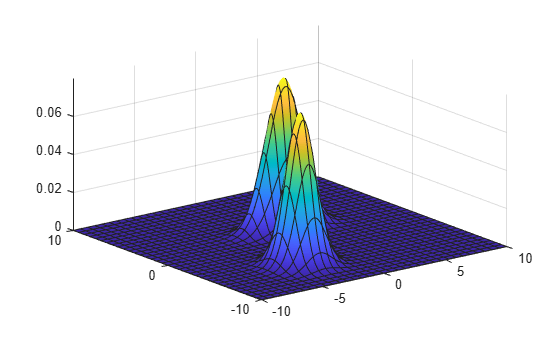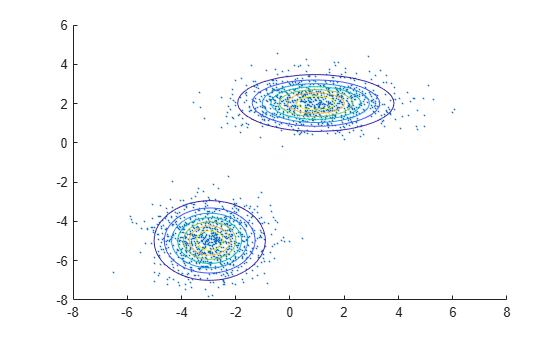# gmdistribution

Create Gaussian mixture model

## Description

A `gmdistribution` object stores a Gaussian mixture distribution, also called a Gaussian mixture model (GMM), which is a multivariate distribution that consists of multivariate Gaussian distribution components. Each component is defined by its mean and covariance. The mixture is defined by a vector of mixing proportions, where each mixing proportion represents the fraction of the population described by a corresponding component.

## Creation

You can create a `gmdistribution` model object in two ways.

• Use the `gmdistribution` function (described here) to create a `gmdistribution` model object by specifying the distribution parameters.

• Use the `fitgmdist` function to fit a `gmdistribution` model object to data given a fixed number of components.

### Syntax

``gm = gmdistribution(mu,sigma)``
``gm = gmdistribution(mu,sigma,p)``

### Description

example

````gm = gmdistribution(mu,sigma)` creates a `gmdistribution` model object using the specified means `mu` and covariances `sigma` with equal mixing proportions.```
````gm = gmdistribution(mu,sigma,p)` specifies the mixing proportions of multivariate Gaussian distribution components.```

### Input Arguments

expand all

Means of multivariate Gaussian distribution components, specified as a k-by-m numeric matrix, where k is the number of components and m is the number of variables in each component. `mu(i,:)` is the mean of component `i`.

Data Types: `single` | `double`

Covariances of multivariate Gaussian distribution components, specified as a numeric vector, matrix, or array.

Given that k is the number of components and m is the number of variables in each component, `sigma` is one of the values in this table.

ValueDescription
m-by-m-by-k array`sigma(:,:,i)` is the covariance matrix of component `i`.
1-by-m-by-k arrayCovariance matrices are diagonal. `sigma(1,:,i)` contains the diagonal elements of the covariance matrix of component `i`.
m-by-m matrixCovariance matrices are the same across components.
1-by-m vectorCovariance matrices are diagonal and the same across components.

Data Types: `single` | `double`

Mixing proportions of mixture components, specified as a numeric vector of length k, where k is the number of components. The default is a row vector of (1/k)s, which sets equal proportions. If `p` does not sum to `1`, `gmdistribution` normalizes it.

Data Types: `single` | `double`

## Properties

expand all

### Distribution Parameters

Means of multivariate Gaussian distribution components, specified as a k-by-m numeric matrix, where k is the number of components and m is the number of variables in each component. `mu(i,:)` is the mean of component `i`.

• If you create a `gmdistribution` object by using the `gmdistribution` function, then the `mu` input argument of `gmdistribution` sets this property.

• If you fit a `gmdistribution` object to data by using the `fitgmdist` function, then `fitgmdist` estimates this property.

Data Types: `single` | `double`

Covariances of multivariate Gaussian distribution components, specified as a numeric vector, matrix, or array.

Given that k is the number of components and m is the number of variables in each component, `Sigma` is one of the values in this table.

ValueDescription
m-by-m-by-k array`Sigma(:,:,i)` is the covariance matrix of component `i`.
1-by-m-by-k arrayCovariance matrices are diagonal. `Sigma(1,:,i)` contains the diagonal elements of the covariance matrix of component `i`.
m-by-m matrixCovariance matrices are the same across components.
1-by-m vectorCovariance matrices are diagonal and the same across components.

• If you create a `gmdistribution` object by using the `gmdistribution` function, then the `sigma` input argument of `gmdistribution` sets this property.

• If you fit a `gmdistribution` object to data by using the `fitgmdist` function, then `fitgmdist` estimates this property.

Data Types: `single` | `double`

Mixing proportions of mixture components, specified as a 1-by-k numeric vector.

• If you create a `gmdistribution` object by using the `gmdistribution` function, then the `p` input argument of `gmdistribution` sets this property.

• If you fit a `gmdistribution` object to data by using the `fitgmdist` function, then `fitgmdist` estimates this property.

Data Types: `single` | `double`

### Distribution Characteristics

Type of covariance matrices, specified as either `'diagonal'` or `'full'`.

• If you create a `gmdistribution` object by using the `gmdistribution` function, then the type of covariance matrices in the `sigma` input argument of `gmdistribution` sets this property.

• If you fit a `gmdistribution` object to data by using the `fitgmdist` function, then the `'CovarianceType'` name-value pair argument of `fitgmdist` sets this property.

Distribution name, specified as ```'gaussian mixture distribution'```.

Number of mixture components, k, specified as a positive integer.

Data Types: `single` | `double`

Number of variables in the multivariate Gaussian distribution components, m, specified as a positive integer.

Data Types: `double`

Flag indicating whether a covariance matrix is shared across mixture components, specified as `true` or `false`.

• If you create a `gmdistribution` object by using the `gmdistribution` function, then the type of covariance matrices in the `sigma` input argument of `gmdistribution` sets this property.

• If you fit a `gmdistribution` object to data by using the `fitgmdist` function, then the `'SharedCovariance'` name-value pair argument of `fitgmdist` sets this property.

Data Types: `logical`

### Properties for Fitted Object

The following properties apply only to a fitted object you create by using `fitgmdist`. The values of these properties are empty if you create a `gmdistribution` object by using the `gmdistribution` function.

Akaike information criterion (AIC), specified as a scalar. `AIC = 2*NlogL + 2*p`, where `NlogL` is the negative loglikelihood (the `NegativeLogLikelihood` property) and `p` is the number of estimated parameters.

AIC is a model selection tool you can use to compare multiple models fit to the same data. AIC is a likelihood-based measure of model fit that includes a penalty for complexity, specifically, the number of parameters. When you compare multiple models, a model with a smaller value of AIC is better.

This property is empty if you create a `gmdistribution` object by using the `gmdistribution` function.

Data Types: `single` | `double`

Bayes information criterion (BIC), specified as a scalar. ```BIC = 2*NlogL + p*log(n)```, where `NlogL` is the negative loglikelihood (the `NegativeLogLikelihood` property), `n` is the number of observations, and `p` is the number of estimated parameters.

BIC is a model selection tool you can use to compare multiple models fit to the same data. BIC is a likelihood-based measure of model fit that includes a penalty for complexity, specifically, the number of parameters. When you compare multiple models, a model with the lowest BIC value is the best fitting model.

This property is empty if you create a `gmdistribution` object by using the `gmdistribution` function.

Data Types: `single` | `double`

Flag indicating whether the Expectation-Maximization (EM) algorithm is converged when fitting a Gaussian mixture model, specified as `true` or `false`.

You can change the optimization options by using the `'Options'` name-value pair argument of `fitgmdist`.

This property is empty if you create a `gmdistribution` object by using the `gmdistribution` function.

Data Types: `logical`

Negative loglikelihood of the fitted Gaussian mixture model given the input data `X` of `fitgmdist`, specified as a scalar.

This property is empty if you create a `gmdistribution` object by using the `gmdistribution` function.

Data Types: `single` | `double`

Number of iterations in the Expectation-Maximization (EM) algorithm, specified as a positive integer.

You can change the optimization options, including the maximum number of iterations allowed, by using the `'Options'` name-value pair argument of `fitgmdist`.

This property is empty if you create a `gmdistribution` object by using the `gmdistribution` function.

Data Types: `double`

Tolerance for posterior probabilities, specified as a nonnegative scalar value in the range `[0,1e-6]`.

The `'ProbabilityTolerance'` name-value pair argument of `fitgmdist` sets this property.

This property is empty if you create a `gmdistribution` object by using the `gmdistribution` function.

Data Types: `single` | `double`

Regularization parameter value, specified as a nonnegative scalar.

The `'RegularizationValue'` name-value pair argument of `fitgmdist` sets this property.

This property is empty if you create a `gmdistribution` object by using the `gmdistribution` function.

Data Types: `single` | `double`

## Object Functions

 `cdf` Cumulative distribution function for Gaussian mixture distribution `cluster` Construct clusters from Gaussian mixture distribution `mahal` Mahalanobis distance to Gaussian mixture component `pdf` Probability density function for Gaussian mixture distribution `posterior` Posterior probability of Gaussian mixture component `random` Random variate from Gaussian mixture distribution

## Examples

collapse all

Create a two-component bivariate Gaussian mixture distribution by using the `gmdistribution` function.

Define the distribution parameters (means and covariances) of two bivariate Gaussian mixture components.

```mu = [1 2;-3 -5]; sigma = cat(3,[2 .5],[1 1]) % 1-by-2-by-2 array```
```sigma = sigma(:,:,1) = 2.0000 0.5000 sigma(:,:,2) = 1 1 ```

The `cat` function concatenates the covariances along the third array dimension. The defined covariance matrices are diagonal matrices. `sigma(1,:,i)` contains the diagonal elements of the covariance matrix of component `i`.

Create a `gmdistribution` object. By default, the `gmdistribution` function creates an equal proportion mixture.

`gm = gmdistribution(mu,sigma)`
```gm = Gaussian mixture distribution with 2 components in 2 dimensions Component 1: Mixing proportion: 0.500000 Mean: 1 2 Component 2: Mixing proportion: 0.500000 Mean: -3 -5 ```

List the properties of the `gm` object.

`properties(gm)`
```Properties for class gmdistribution: NumVariables DistributionName NumComponents ComponentProportion SharedCovariance NumIterations RegularizationValue NegativeLogLikelihood CovarianceType mu Sigma AIC BIC Converged ProbabilityTolerance ```

You can access these properties by using dot notation. For example, access the `ComponentProportion` property, which represents the mixing proportions of mixture components.

`gm.ComponentProportion`
```ans = 1×2 0.5000 0.5000 ```

A `gmdistribution` object has properties that apply only to a fitted object. The fitted object properties are `AIC`, `BIC`, `Converged`, `NegativeLogLikelihood`, `NumIterations`, `ProbabilityTolerance`, and `RegularizationValue`. The values of the fitted object properties are empty if you create an object by using the `gmdistribution` function and specifying distribution parameters. For example, access the `NegativeLogLikelihood` property by using dot notation.

`gm.NegativeLogLikelihood`
```ans = [] ```

After you create a `gmdistribution` object, you can use the object functions. Use `cdf` and `pdf` to compute the values of the cumulative distribution function (cdf) and the probability density function (pdf). Use `random` to generate random vectors. Use `cluster`, `mahal`, and `posterior` for cluster analysis.

Visualize the object by using `pdf` and `fsurf`.

```gmPDF = @(x,y) arrayfun(@(x0,y0) pdf(gm,[x0 y0]),x,y); fsurf(gmPDF,[-10 10])```Generate random variates that follow a mixture of two bivariate Gaussian distributions by using the `mvnrnd` function. Fit a Gaussian mixture model (GMM) to the generated data by using the `fitgmdist` function.

Define the distribution parameters (means and covariances) of two bivariate Gaussian mixture components.

```mu1 = [1 2]; % Mean of the 1st component sigma1 = [2 0; 0 .5]; % Covariance of the 1st component mu2 = [-3 -5]; % Mean of the 2nd component sigma2 = [1 0; 0 1]; % Covariance of the 2nd component```

Generate an equal number of random variates from each component, and combine the two sets of random variates.

```rng('default') % For reproducibility r1 = mvnrnd(mu1,sigma1,1000); r2 = mvnrnd(mu2,sigma2,1000); X = [r1; r2];```

The combined data set `X` contains random variates following a mixture of two bivariate Gaussian distributions.

Fit a two-component GMM to `X`.

`gm = fitgmdist(X,2)`
```gm = Gaussian mixture distribution with 2 components in 2 dimensions Component 1: Mixing proportion: 0.500000 Mean: -2.9617 -4.9727 Component 2: Mixing proportion: 0.500000 Mean: 0.9539 2.0261 ```

List the properties of the `gm` object.

`properties(gm)`
```Properties for class gmdistribution: NumVariables DistributionName NumComponents ComponentProportion SharedCovariance NumIterations RegularizationValue NegativeLogLikelihood CovarianceType mu Sigma AIC BIC Converged ProbabilityTolerance ```

You can access these properties by using dot notation. For example, access the `NegativeLogLikelihood` property, which represents the negative loglikelihood of the data `X` given the fitted model.

`gm.NegativeLogLikelihood`
```ans = 7.0584e+03 ```

After you create a `gmdistribution` object, you can use the object functions. Use `cdf` and `pdf` to compute the values of the cumulative distribution function (cdf) and the probability density function (pdf). Use `random` to generate random variates. Use `cluster`, `mahal`, and `posterior` for cluster analysis.

Plot `X` by using `scatter`. Visualize the fitted model `gm` by using `pdf` and `fcontour`.

```scatter(X(:,1),X(:,2),10,'.') % Scatter plot with points of size 10 hold on gmPDF = @(x,y) arrayfun(@(x0,y0) pdf(gm,[x0 y0]),x,y); fcontour(gmPDF,[-8 6])```McLachlan, G., and D. Peel. Finite Mixture Models. Hoboken, NJ: John Wiley & Sons, Inc., 2000.